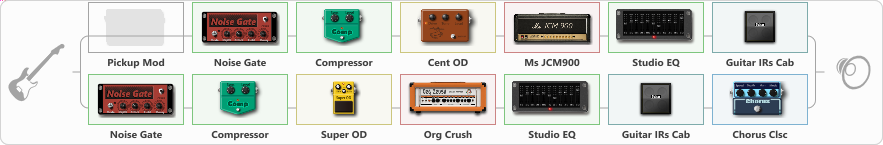# Slipknot's Iowa Tone

Discussion in 'ToneLib-GFX presets' started by clever1, Jun 4, 2021.

1. Slipknot's Iowa Tone

Preset name: Mick Thompson and Jim Tone combined

This tone was made specifically for EMGs or Humbuckers. If you do not have a humbucker, you can turn on the pick up mod and also get a booster + OD before the splitter and you will get a good tone regardless. With that out the way, I might upload a video showcasing the tone, but I will update the link if I do. In the meantime I will just leave my channel if anyone is interested in my tones

Related video:

Effects chain:Effect: "Splitter" (Dynamics / Filter)
{
"A-Bypass" = Off
"A-Pan" = -100
"A-Level" = 53
"B-Bypass" = Off
"B-Pan" = 100
"B-Level" = 52

'A' branch:
{

Effect: "Pickup Mod" (Dynamics / Filter), active - "no"
{
"Mode" = Single->Humbacker
"Tone" = 64
}

Effect: "Noise Gate" (Dynamics / Filter), active - "yes"
{
"Mode" = Manual
"Depth" = 14
"Threshold" = 73
"Attack" = 0
"Hold" = 5
"Decay" = 250
}

Effect: "Compressor" (Dynamics / Filter), active - "yes"
{
"Sense" = 30
"Level" = 70
}

Effect: "Cent OD" (Overdrive / Distortion), active - "yes"
{
"Drive" = 65
"Tone" = 76
"Level" = 64
}

Effect: "Ms JCM900" (Amp simulators), active - "yes"
{
"Gain" = 79
"Bass" = 34
"Middle" = 60
"Treble" = 40
"Presence" = 55
"Master" = 100
"Level (dB)" = 0
}

Effect: "Studio EQ" (Dynamics / Filter), active - "yes"
{
"31 Hz" = 1
"62 Hz" = 2
"125 Hz" = 0
"250 Hz" = 1
"500 Hz" = 1
"1 kHz" = 2
"2 kHz" = -2
"4 kHz" = -3
"8 kHz" = -4
"16 kHz" = -10
"Level (dB)" = 0
}

Effect: "Guitar IRs Cab" (Cabinets), active - "yes"
{
"Model" = Marshall 1960A (4x12")
"Mic Position" = Center
"Mic Distance" = Near
"Low Cut (Hz)" = 149
"Hi Cut (kHz)" = 18.0
"Mix" = 100
"Level (dB)" = -1
}
}
'B' branch:
{

Effect: "Noise Gate" (Dynamics / Filter), active - "yes"
{
"Mode" = Manual
"Depth" = 8
"Threshold" = 76
"Attack" = 0
"Hold" = 5
"Decay" = 322
}

Effect: "Compressor" (Dynamics / Filter), active - "yes"
{
"Sense" = 45
"Level" = 2
}

Effect: "Super OD" (Overdrive / Distortion), active - "yes"
{
"Drive" = 72
"Tone" = 80
"Level" = 91
}

Effect: "Org Crush" (Amp simulators), active - "yes"
{
"Gain" = 73
"Bass" = 72
"Middle" = 41
"Treble" = 54
"Presence" = 54
"Master" = 80
"Level (dB)" = 0
}

Effect: "Studio EQ" (Dynamics / Filter), active - "yes"
{
"31 Hz" = 0
"62 Hz" = 0
"125 Hz" = 0
"250 Hz" = 0
"500 Hz" = 0
"1 kHz" = 0
"2 kHz" = -1
"4 kHz" = -2
"8 kHz" = -1
"16 kHz" = -6
"Level (dB)" = 0
}

Effect: "Guitar IRs Cab" (Cabinets), active - "yes"
{
"Model" = Orange PPC (4x12")
"Mic Position" = Edge
"Mic Distance" = Far
"Low Cut (Hz)" = 110
"Hi Cut (kHz)" = 15.0
"Mix" = 100
"Level (dB)" = -2
}

Effect: "Chorus Clsc" (Modulation / Sfx), active - "yes"
{
"Speed" = 0.1
"Depth" = 0
"Center" = 1.0
"Mode" = Stereo
}
}
}

Note: You will need to download and install the ToneLib-GFX software to use the preset.

#### Attached Files:

• ###### Slipknots_Iowa_Tone.tlgfx
File size:
1.5 KB
Views:
2,747
Eikyty, SEMENOV, Nazaroff and 4 others like this.